# Tutorial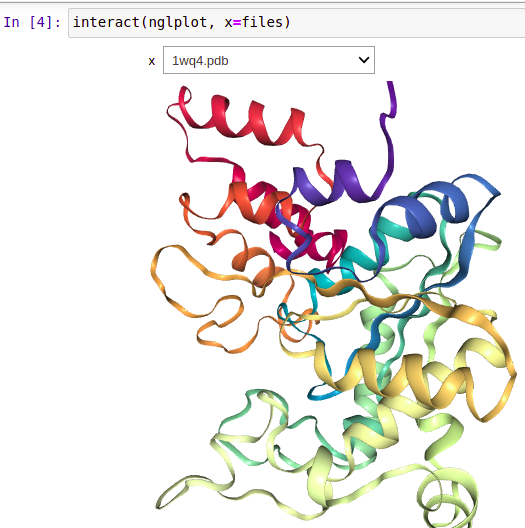## Visualizing 3D Protein Structures and Exporting Into images Using Jupyter Notebook.

The Jupyter Notebook is best suited for doing as well as documenting empirical research. It can contain text, code images, videos, interactive content, and also nicely typesetting equations. This makes anyone doing simulations or empirical research prototype their work and share it with their colleagues. In this blog post, I am going to show how …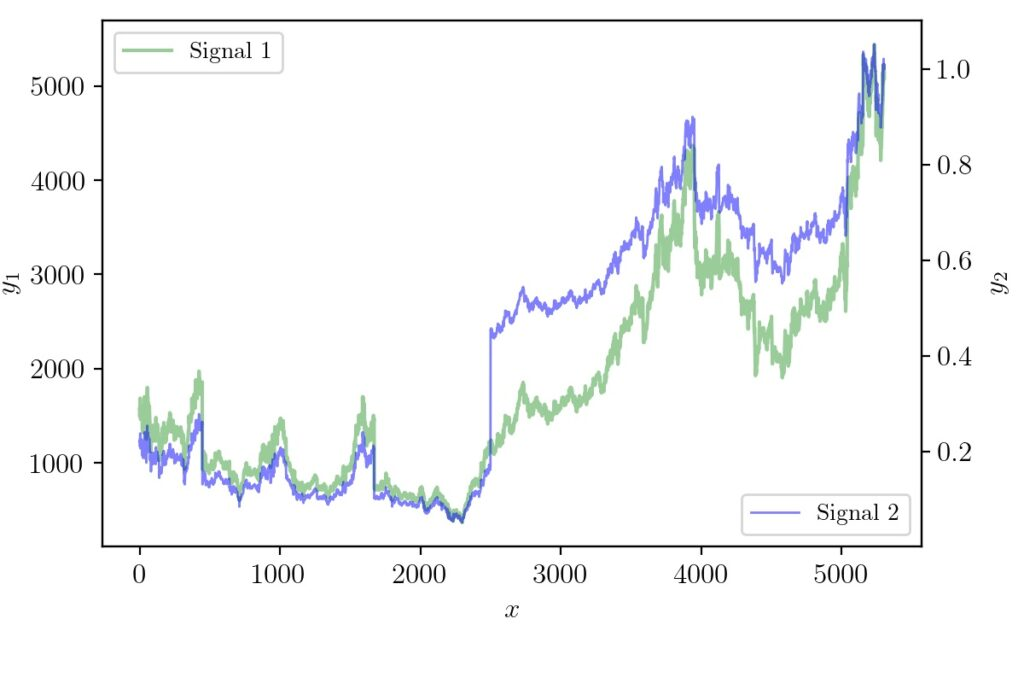## Python Matplotlib Tip: Overlapping Curves and Multiple Axes

In STEM fields it is often required to communicate ideas using graphs or infographics. Many times the dimensionality of data will be higher and require clever hacks to represent so that the reader gets the idea quickly. There have been systematic studies on how to do data visualization effectively. For data scientists or machine learning …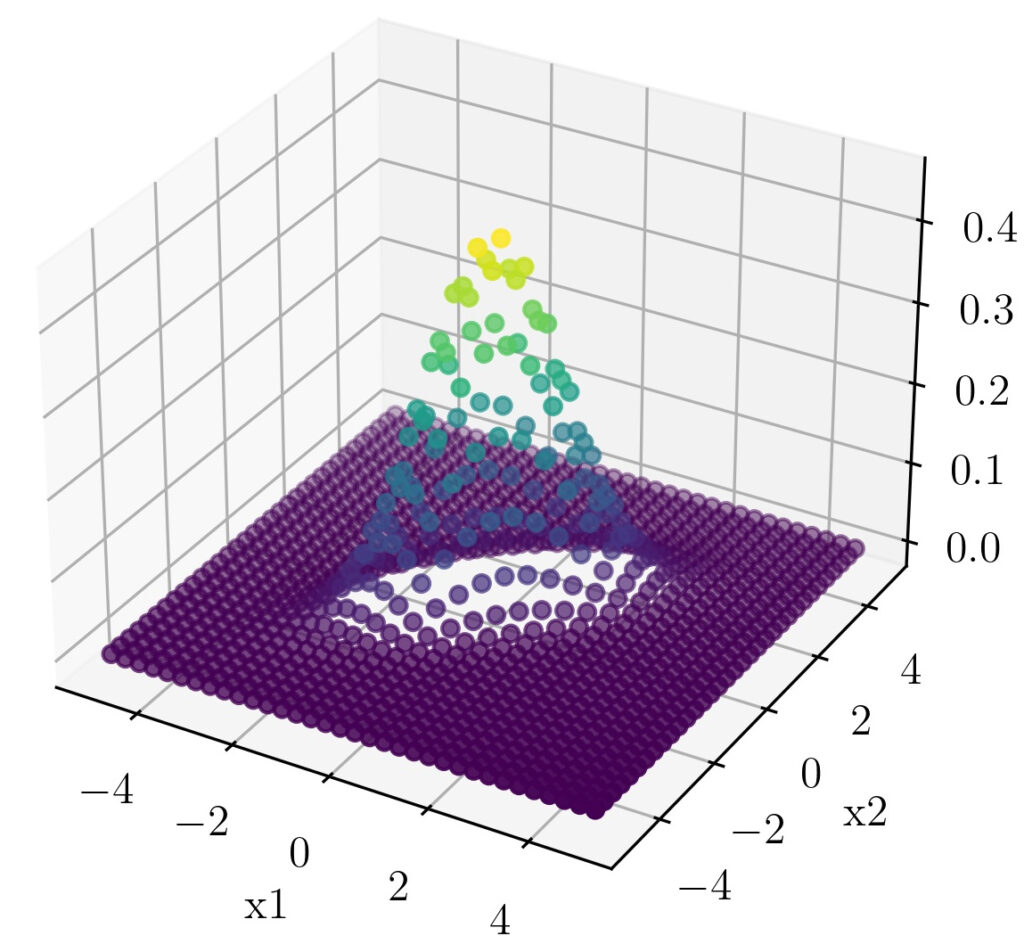## Hacking the Bivariate Gaussian Distribution

In one of our earlier posts, we have seen how we can visually relate the parts of the one-dimensional Gaussian distribution equation. In this post, we will follow the same strategy to understand the terms that comes up with a Multivariable Gaussian distribution. We will focus on the Bivariate Gaussian distribution as distributions of higher-order …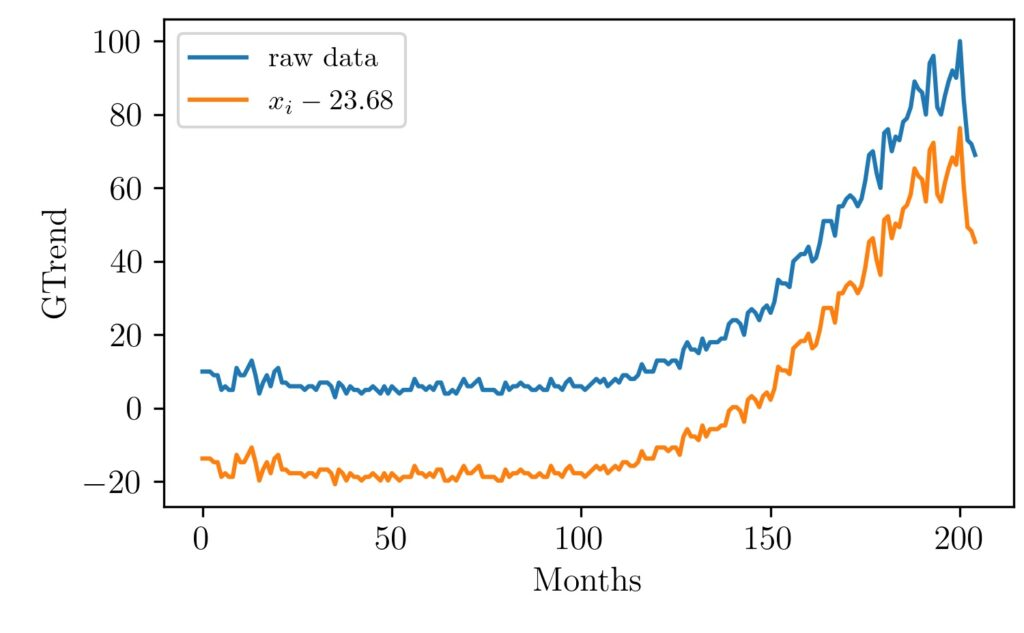## Visualizing MinMax Scaling

Normalization of vectors, an array of values, signals is often used as a preprocessing step before many algorithms. For example, in machine learning, some types of algorithms are prone to different inherent scales of features. In such situations normalization is done to give the same weightage to all features before passing it to the algorithm …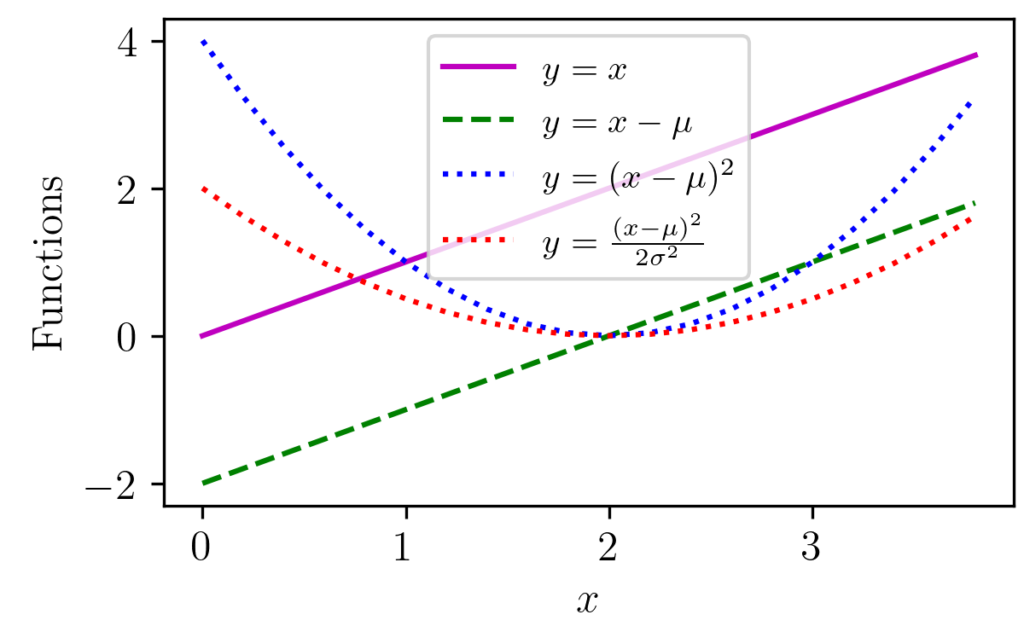## Gaussian Distribution Explained Visually

Gaussian distribution appears in various parts of science and engineering. Apart from a distribution often appear in nature, it has got important properties such as its relation to Central Limit Theorem (CLT). The figure above shows one-dimensional Gaussian distributions of various mean and variance values. Libraries like NumPy provide functions that can return Gaussian distribution …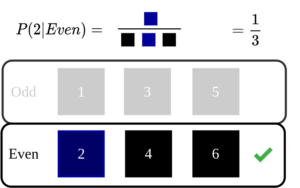## An Intuitive Explanation of Naive Bayes Classifier

Introduction In this post, let’s take a look at the intuition behind Naive Bayes Classifier used in machine learning. Naive Bayes classifier is one of the basic algorithms often encountered in machine learning applications. If linear regression was based on concepts from linear algebra and calculus, naive Bayes classifier mostly backed up by probability theory. …